### Showing:

 Annotations Attributes Diagrams Facets Instances Model Properties Source Used by
Main schema spase-2.2.2.xsd
Namespace http://www.spase-group.org/data/schema
Properties
 attribute form default unqualified element form default qualified version 2.2.2
Element spase:Spase
Namespace http://www.spase-group.org/data/schema
Diagram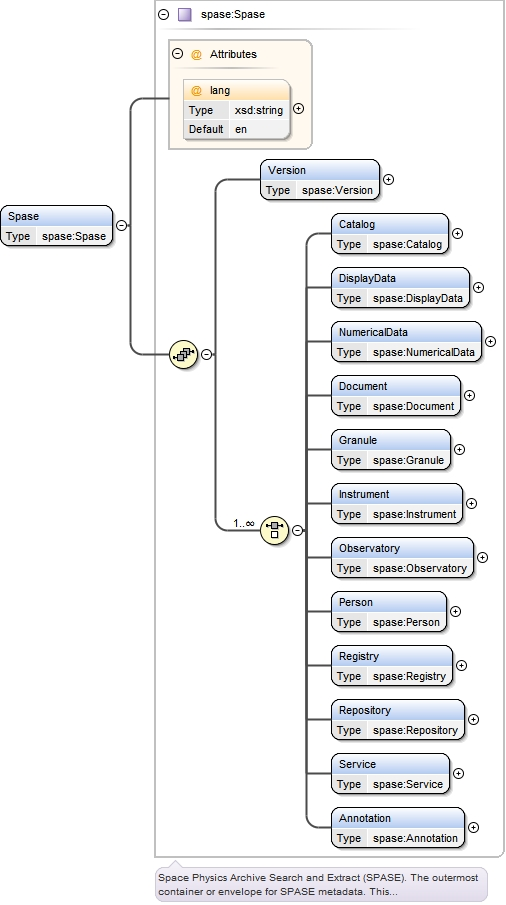Type spase:Spase
Properties
 content complex
Model
Children spase:Annotation, spase:Catalog, spase:DisplayData, spase:Document, spase:Granule, spase:Instrument, spase:NumericalData, spase:Observatory, spase:Person, spase:Registry, spase:Repository, spase:Service, spase:Version
Instance
 ``` {1,1} {1,1} {1,1} {1,1} {1,1} {1,1} {1,1} {1,1} {1,1} {1,1} {1,1} {1,1} {1,1} ```
Attributes
QName Type Default Use
lang xsd:string en optional
Source
 ``
Element spase:Spase / spase:Version
Namespace http://www.spase-group.org/data/schema
Diagram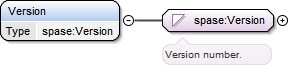Type spase:Version
Properties
 content simple minOccurs 1 maxOccurs 1
Facets
 enumeration 2.2.2
Source
 ``
Element spase:Spase / spase:Catalog
Namespace http://www.spase-group.org/data/schema
Diagram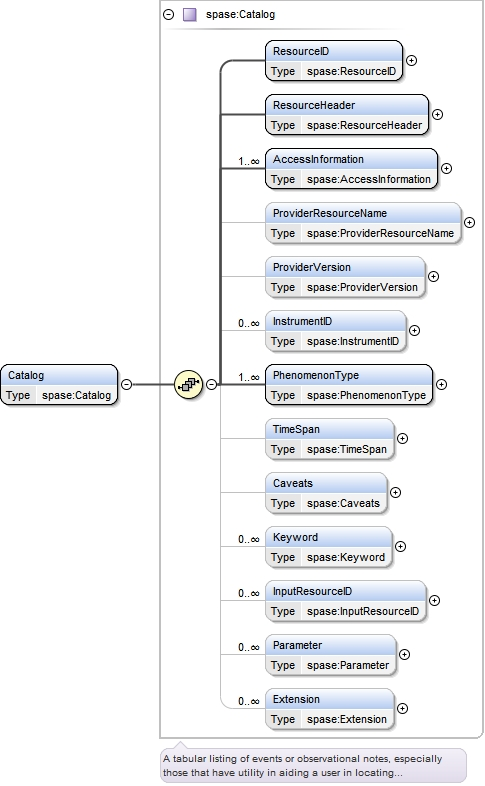Type spase:Catalog
Properties
 content complex
Model
Children spase:AccessInformation, spase:Caveats, spase:Extension, spase:InputResourceID, spase:InstrumentID, spase:Keyword, spase:Parameter, spase:PhenomenonType, spase:ProviderResourceName, spase:ProviderVersion, spase:ResourceHeader, spase:ResourceID, spase:TimeSpan
Instance
 ``` {1,1} {1,1} {1,unbounded} {0,1} {0,1} {0,unbounded} {1,unbounded} {0,1} {0,1} {0,unbounded} {0,unbounded} {0,unbounded} {0,unbounded} ```
Source
 ``
Element spase:Catalog / spase:ResourceID
Namespace http://www.spase-group.org/data/schema
DiagramType spase:ResourceID
Properties
 content simple minOccurs 1 maxOccurs 1
Source
 ``
Namespace http://www.spase-group.org/data/schema
Diagram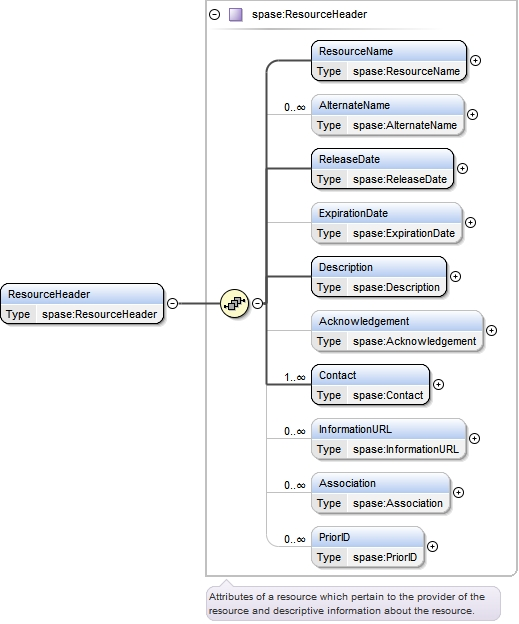Properties
 content complex minOccurs 1 maxOccurs 1
Model
Children spase:Acknowledgement, spase:AlternateName, spase:Association, spase:Contact, spase:Description, spase:ExpirationDate, spase:InformationURL, spase:PriorID, spase:ReleaseDate, spase:ResourceName
Instance
 ``` {1,1} {0,unbounded} {1,1} {0,1} {1,1} {0,1} {1,unbounded} {0,unbounded} {0,unbounded} {0,unbounded} ```
Source
 ``
Namespace http://www.spase-group.org/data/schema
DiagramType spase:ResourceName
Properties
 content simple minOccurs 1 maxOccurs 1
Source
 ``
Namespace http://www.spase-group.org/data/schema
DiagramType spase:AlternateName
Properties
 content simple minOccurs 0 maxOccurs unbounded
Source
 ``
Namespace http://www.spase-group.org/data/schema
DiagramType spase:ReleaseDate
Properties
 content simple minOccurs 1 maxOccurs 1
Source
 ``
Namespace http://www.spase-group.org/data/schema
Diagram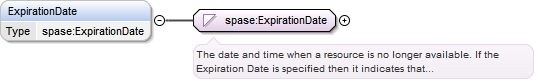Type spase:ExpirationDate
Properties
 content simple minOccurs 0 maxOccurs 1
Source
 ``
Namespace http://www.spase-group.org/data/schema
DiagramType spase:Description
Properties
 content simple minOccurs 1 maxOccurs 1
Source
 ``
Namespace http://www.spase-group.org/data/schema
DiagramType spase:Acknowledgement
Properties
 content simple minOccurs 0 maxOccurs 1
Source
 ``
Namespace http://www.spase-group.org/data/schema
Diagram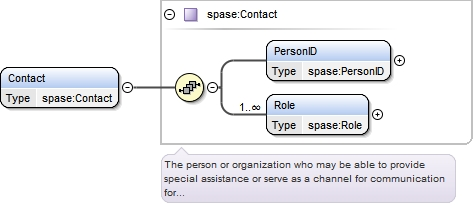Type spase:Contact
Properties
 content complex minOccurs 1 maxOccurs unbounded
Model
Children spase:PersonID, spase:Role
Instance
 ``` {1,1} {1,unbounded} ```
Source
 ``
Element spase:Contact / spase:PersonID
Namespace http://www.spase-group.org/data/schema
DiagramType spase:PersonID
Properties
 content simple minOccurs 1 maxOccurs 1
Source
 ``
Element spase:Contact / spase:Role
Namespace http://www.spase-group.org/data/schema
DiagramType spase:Role
Properties
 content simple minOccurs 1 maxOccurs unbounded
Facets
enumeration ArchiveSpecialist
 `An individual who is an expert on a collection of resources and may also be knowledgeable of the phenomenon and related physics represented by the resources. This includes librarians, curators, archive scientists and other experts.`
enumeration CoInvestigator
 `An individual who is a scientific peer and major participant in an investigation.`
enumeration Contributor
 `An entity responsible for making contributions to the content of the resource.`
enumeration DataProducer
 `An individual who generated the resource and is familiar with its provenance.`
enumeration DeputyPI
 `An individual who is an administrative or scientific leader for an investigation operating under the supervision of a Principal Investigator.`
enumeration FormerPI
 `An individual who had served as the administrative and scientific lead for an investigation, but no longer assumes that role.`
enumeration GeneralContact
 `An individual who can provide information on a range of subjects or who can direct you to a domain expert.`
 `An individual who can affect a change in the metadata describing a resource.`
enumeration PrincipalInvestigator
 `An individual who is the administrative and scientific lead for an investigation.`
enumeration ProjectScientist
 `An individual who is an expert in the phenomenon and related physics explored by the project. A project scientist may also have a managerial role within the project.`
enumeration Publisher
 `An individual, organization, institution or government department responsible for the production and dissemination of a document.`
enumeration Scientist
 `An individual who is an expert in the phenomenon and related physics represented by the resource.`
 `An individual who is the designated leader of an investigation.`
enumeration TeamMember
 `An individual who is a major participant in an investigation.`
enumeration TechnicalContact
 `An individual who can provide specific information with regard to the resource or supporting software`
Source
 ``
Namespace http://www.spase-group.org/data/schema
Diagram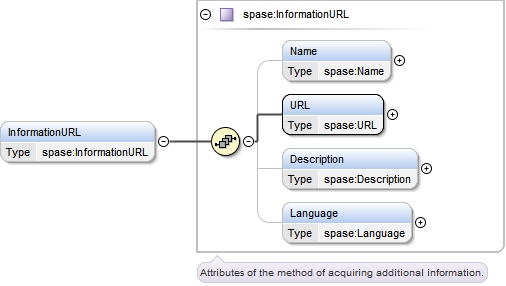Type spase:InformationURL
Properties
 content complex minOccurs 0 maxOccurs unbounded
Model
Children spase:Description, spase:Language, spase:Name, spase:URL
Instance
 ``` {0,1} {1,1} {0,1} {0,1} ```
Source
 ``
Element spase:InformationURL / spase:Name
Namespace http://www.spase-group.org/data/schema
DiagramType spase:Name
Properties
 content simple minOccurs 0 maxOccurs 1
Source
 ``
Element spase:InformationURL / spase:URL
Namespace http://www.spase-group.org/data/schema
DiagramType spase:URL
Properties
 content simple minOccurs 1 maxOccurs 1
Source
 ``
Element spase:InformationURL / spase:Description
Namespace http://www.spase-group.org/data/schema
DiagramType spase:Description
Properties
 content simple minOccurs 0 maxOccurs 1
Source
 ``
Element spase:InformationURL / spase:Language
Namespace http://www.spase-group.org/data/schema
Diagram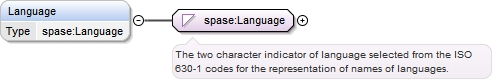Type spase:Language
Properties
 content simple minOccurs 0 maxOccurs 1
Source
 ``
Namespace http://www.spase-group.org/data/schema
Diagram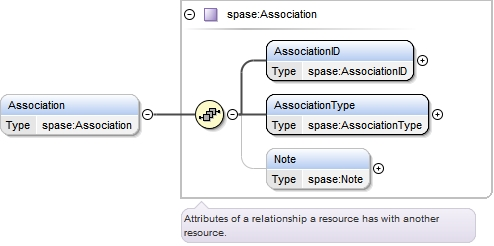Type spase:Association
Properties
 content complex minOccurs 0 maxOccurs unbounded
Model
Children spase:AssociationID, spase:AssociationType, spase:Note
Instance
 ``` {1,1} {1,1} {0,1} ```
Source
 ``
Element spase:Association / spase:AssociationID
Namespace http://www.spase-group.org/data/schema
Diagram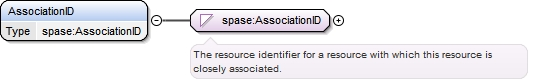Type spase:AssociationID
Properties
 content simple minOccurs 1 maxOccurs 1
Source
 ``
Element spase:Association / spase:AssociationType
Namespace http://www.spase-group.org/data/schema
Diagram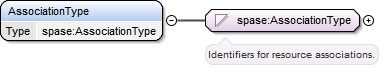Type spase:AssociationType
Properties
 content simple minOccurs 1 maxOccurs 1
Facets
enumeration ChildEventOf
 `A descendant or caused by another resource.`
enumeration DerivedFrom
 `A transformed or altered version of a resource instance.`
enumeration ObservedBy
 `Detected or originating from another resource.`
enumeration Other
 `Not classified with more specific terms. The context of its usage may be described in related text.`
enumeration PartOf
 `A portion of a larger resource.`
enumeration RevisionOf
 `A modified version of a resource instance.`
Source
 ``
Element spase:Association / spase:Note
Namespace http://www.spase-group.org/data/schema
DiagramType spase:Note
Properties
 content simple minOccurs 0 maxOccurs 1
Source
 ``
Namespace http://www.spase-group.org/data/schema
DiagramType spase:PriorID
Properties
 content simple minOccurs 0 maxOccurs unbounded
Source
 ``
Element spase:Catalog / spase:AccessInformation
Namespace http://www.spase-group.org/data/schema
Diagram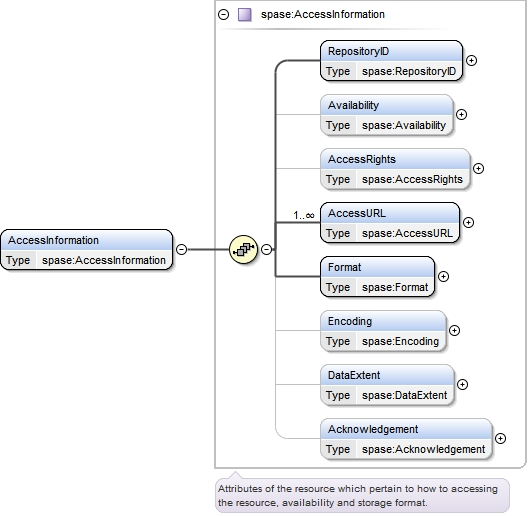Type spase:AccessInformation
Properties
 content complex minOccurs 1 maxOccurs unbounded
Model
Children spase:AccessRights, spase:AccessURL, spase:Acknowledgement, spase:Availability, spase:DataExtent, spase:Encoding, spase:Format, spase:RepositoryID
Instance
 ``` {1,1} {0,1} {0,1} {1,unbounded} {1,1} {0,1} {0,1} {0,1} ```
Source
 ``
Element spase:AccessInformation / spase:RepositoryID
Namespace http://www.spase-group.org/data/schema
DiagramType spase:RepositoryID
Properties
 content simple minOccurs 1 maxOccurs 1
Source
 ``
Element spase:AccessInformation / spase:Availability
Namespace http://www.spase-group.org/data/schema
DiagramType spase:Availability
Properties
 content simple minOccurs 0 maxOccurs 1
Facets
enumeration Offline
 `Not directly accessible electronically. This includes resources which may to be moved to an on-line status in response to a given request.`
enumeration Online
 `Directly accessible electronically.`
Source
 ``
Element spase:AccessInformation / spase:AccessRights
Namespace http://www.spase-group.org/data/schema
DiagramType spase:AccessRights
Properties
 content simple minOccurs 0 maxOccurs 1
Facets
enumeration Open
 `Access is granted to everyone.`
enumeration Restricted
 `Access to the product is regulated and requires some form of identification.`
Source
 ``
Element spase:AccessInformation / spase:AccessURL
Namespace http://www.spase-group.org/data/schema
Diagram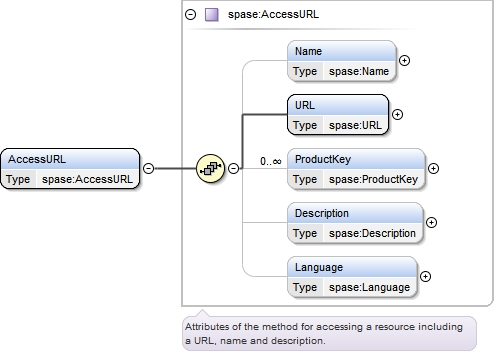Type spase:AccessURL
Properties
 content complex minOccurs 1 maxOccurs unbounded
Model
Children spase:Description, spase:Language, spase:Name, spase:ProductKey, spase:URL
Instance
 ``` {0,1} {1,1} {0,unbounded} {0,1} {0,1} ```
Source
 ``
Element spase:AccessURL / spase:Name
Namespace http://www.spase-group.org/data/schema
DiagramType spase:Name
Properties
 content simple minOccurs 0 maxOccurs 1
Source
 ``
Element spase:AccessURL / spase:URL
Namespace http://www.spase-group.org/data/schema
DiagramType spase:URL
Properties
 content simple minOccurs 1 maxOccurs 1
Source
 ``
Element spase:AccessURL / spase:ProductKey
Namespace http://www.spase-group.org/data/schema
DiagramType spase:ProductKey
Properties
 content simple minOccurs 0 maxOccurs unbounded
Source
 ``
Element spase:AccessURL / spase:Description
Namespace http://www.spase-group.org/data/schema
DiagramType spase:Description
Properties
 content simple minOccurs 0 maxOccurs 1
Source
 ``
Element spase:AccessURL / spase:Language
Namespace http://www.spase-group.org/data/schema
DiagramType spase:Language
Properties
 content simple minOccurs 0 maxOccurs 1
Source
 ``
Element spase:AccessInformation / spase:Format
Namespace http://www.spase-group.org/data/schema
DiagramType spase:Format
Properties
 content simple minOccurs 1 maxOccurs 1
Facets
enumeration AVI
 `Audio Video Interleave (AVI) a digital format for movies that conforms to the Microsoft Windows Resource Interchange File Format (RIFF).`
enumeration Binary
 `A direct representation of the bits which may be stored in memory on a computer.`
enumeration CDF
 `Common Data Format (CDF). A binary storage format developed at Goddard Space Flight Center (GSFC).`
enumeration CEF
 `Cluster Exchange Format (CEF) is a self-documenting ASCII format designed for the exchange of data. There are two versions of CEF which are not totally compatible.`
enumeration CEF1
 `Cluster Exchange Format (CEF), version 1, is a self-documenting ASCII format designed for the exchange of data. The metadata contains information compatible with the ISTP recommendations for CDF.`
enumeration CEF2
 `Cluster Exchange Format (CEF), version 2, is a self-documenting ASCII format designed for the exchange of data and introduced for Cluster Active Archive. Compared to version 1, the metadata description of vectors and tensors is different.`
enumeration Excel
 `A Microsoft spreadsheet format used to hold a variety of data in tables which can include calculations.`
enumeration FITS
 `Flexible Image Transport System (FITS) is a digital format primarily designed to store scientific data sets consisting of multi-dimensional arrays (1-D spectra, 2-D images or 3-D data cubes) and 2-dimensional tables containing rows and columns of data.`
enumeration GIF
 `Graphic Interchange Format (GIF) first introduced in 1987 by CompuServe. GIF uses LZW compression and images are limited to 256 colours.`
enumeration HDF
 `Hierarchical Data Format`
enumeration HDF4
 `Hierarchical Data Format, Version 4`
enumeration HDF5
 `Hierarchical Data Format, Version 5`
enumeration HTML
 `A text file containing structured information represented in the HyperText Mark-up Language (HTML). See `
enumeration Hardcopy
 `A permanent reproduction, or copy in the form of a physical object, of any media suitable for direct use by a person.`
enumeration Hardcopy.Film
 `An image recording medium on which usually a negative analog image is registered. A positive image can be recovered or reproduced from film, which is usually made of flexible materials for ease of storage and transportation.`
enumeration Hardcopy.Microfiche
 `A sheet of microfilm on which many pages of material have been photographed; a magnification system is used to read the material.`
enumeration Hardcopy.Microfilm
 `Film rolls on which materials are photographed at greatly reduced size; a magnification system is used to read the material.`
enumeration Hardcopy.Photograph
 `An image (positive or negative) registered on a piece of photo-sensitive paper`
enumeration Hardcopy.PhotographicPlate
 `A rigid (typically glass) medium that functions like film. Its rigidity is for guarding against image distortion due to medium deformation (caused by heat and humidity). Photographic plates are often used for astronomical photography.`
enumeration Hardcopy.Print
 `A sheet of any written or printed material which may include notes or graphics. Multiple printed pages may be bound into a manuscript or book.`
enumeration IDFS
 `Instrument Data File Set (IDFS) is a set of files written in a prescribed format which contain data, timing data, and meta-data. IDFS was developed at Southwest Research Institute (SwRI).`
enumeration IDL
 `Interactive Data Language (IDL) save set. IDL is a proprietary format.`
enumeration JPEG
 `A binary format for still images defined by the Joint Photographic Experts Group`
enumeration MATLAB_4
 `MATLAB Workspace save set, version 4. MAT-files are double-precision, binary, MATLAB format files. MATLAB is a proprietary product of The MathWorks.`
enumeration MATLAB_6
 `MATLAB Workspace save set, version 6. MAT-files are double-precision, binary, MATLAB format files. MATLAB is a proprietary product of The MathWorks.`
enumeration MATLAB_7
 `MATLAB Workspace save set, version 7. MAT-files are double-precision, binary, MATLAB format files. Version 7 includes data compression and Unicode encoding. MATLAB is a proprietary product of The MathWorks.`
enumeration MPEG
 `A digital format for movies defined by the Motion Picture Experts Group`
enumeration NCAR
 `The National Center for Atmospheric Research (NCAR) format. A complete description of that standard is given in appendix C of the Report on Establishment & Operation of the Incoherent- Scatter Data Base, dated August 23, 1984, obtainable from NCAR, P.O. Box 3000 Boulder, Colorado 80307-3000.`
enumeration NetCDF
 `Unidata Program Centers Network Common Data Form (NetCDF). A self-describing portable data format for array-oriented data access. See `
enumeration PDF
 `A document expressed in the Portable Document Format (PDF) as defined by Adobe.`
enumeration PNG
 `A digital format for still images. Portable Network Graphics (PNG)`
enumeration Postscript
 `A page description programming language created by Adobe Systems Inc. that is a device-independent industry standard for representing text and graphics.`
enumeration QuickTime
 `A format for digital movies, as defined by Apple Computer. See `
enumeration TIFF
 `A binary format for still pictures. Tagged Image Format File (TIFF). Originally developed by Aldus and now controlled by Adobe.`
enumeration Text
 `A sequence of characters which may have an imposed structure or organization.`
enumeration Text.ASCII
 `A sequence of characters that adheres to American Standard Code for Information Interchange (ASCII) which is an 7-bit character-coding scheme.`
enumeration Text.Unicode
 `Text in multi-byte Unicode format.`
enumeration UDF
 `Universal Data Format (UDF). The Optical Technology Storage Associations Universal Disk Format, based on ISO 13346. See `
enumeration VOTable
 `A proposed IVOA standard designed as a flexible storage and exchange format for tabular data.`
enumeration XML
 `eXtensible Mark-up Language (XML). A structured format for representing information. See `
Source
 ``
Element spase:AccessInformation / spase:Encoding
Namespace http://www.spase-group.org/data/schema
Diagram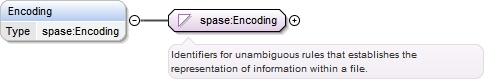Type spase:Encoding
Properties
 content simple minOccurs 0 maxOccurs 1
Facets
enumeration ASCII
 `A sequence of characters that adheres to American Standard Code for Information Interchange (ASCII) which is an 7-bit character-coding scheme.`
enumeration BZIP2
 `An open standard algorithm by Julian Seward using Burrows-Wheeler block sorting and Huffman coding. See `
enumeration Base64
 `A data encoding scheme whereby binary-encoded data is converted to printable ASCII characters. It is defined as a MIME content transfer encoding for use in Internet e-mail. The only characters used are the upper- and lower-case Roman alphabet characters (A-Z, a-z), the numerals (0-9), and the + and / symbols, with the = symbol as a special suffix (padding) code.`
enumeration GZIP
 `An open standard algorithm distributed by GHU based on LZ77 and Huffman coding. See or `
enumeration None
 `A lack or absence of anything.`
enumeration S3_BUCKET
 `A container of objects that comply with the Amazon Simple Storage Service (S3) specifications. A bucket has a unique, user-assigned key (name). A bucket can contain any number of objects with an aggregate size of 5 gigabytes. A bucket may be accompanied by up to 2 kilobytes of metadata.`
enumeration TAR
 `A file format used to collate collections of files into one larger file, for distribution or archiving, while preserving file system information such as user and group permissions, dates, and directory structures. The format was standardized by POSIX.1-1988 and later POSIX.1-2001.`
enumeration Unicode
 `Text in multi-byte Unicode format.`
enumeration ZIP
 `An open standard for compression which is a variation of the LZW method and was originally used in the PKZIP utility.`
Source
 ``
Element spase:AccessInformation / spase:DataExtent
Namespace http://www.spase-group.org/data/schema
Diagram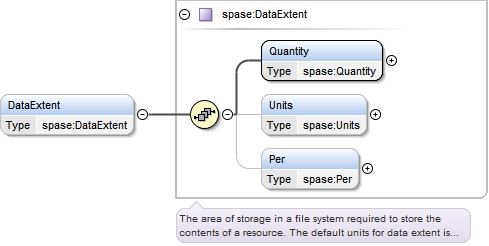Type spase:DataExtent
Properties
 content complex minOccurs 0 maxOccurs 1
Model
Children spase:Per, spase:Quantity, spase:Units
Instance
 ``` {1,1} {0,1} {0,1} ```
Source
 ``
Element spase:DataExtent / spase:Quantity
Namespace http://www.spase-group.org/data/schema
DiagramType spase:Quantity
Properties
 content simple minOccurs 1 maxOccurs 1
Source
 ``
Element spase:DataExtent / spase:Units
Namespace http://www.spase-group.org/data/schema
Diagram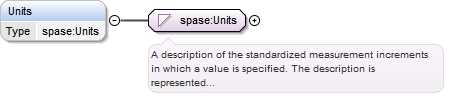Type spase:Units
Properties
 content simple minOccurs 0 maxOccurs 1
Source
 ``
Element spase:DataExtent / spase:Per
Namespace http://www.spase-group.org/data/schema
DiagramType spase:Per
Properties
 content simple minOccurs 0 maxOccurs 1
Source
 ``
Element spase:AccessInformation / spase:Acknowledgement
Namespace http://www.spase-group.org/data/schema
DiagramType spase:Acknowledgement
Properties
 content simple minOccurs 0 maxOccurs 1
Source
 ``
Element spase:Catalog / spase:ProviderResourceName
Namespace http://www.spase-group.org/data/schema
DiagramType spase:ProviderResourceName
Properties
 content simple minOccurs 0 maxOccurs 1
Source
 ``
Element spase:Catalog / spase:ProviderVersion
Namespace http://www.spase-group.org/data/schema
DiagramType spase:ProviderVersion
Properties
 content simple minOccurs 0 maxOccurs 1
Source
 ``
Element spase:Catalog / spase:InstrumentID
Namespace http://www.spase-group.org/data/schema
Diagram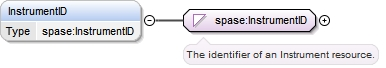Type spase:InstrumentID
Properties
 content simple minOccurs 0 maxOccurs unbounded
Source
 ``
Element spase:Catalog / spase:PhenomenonType
Namespace http://www.spase-group.org/data/schema
Diagram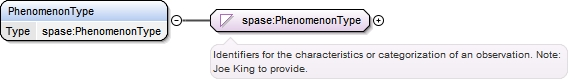Type spase:PhenomenonType
Properties
 content simple minOccurs 1 maxOccurs unbounded
Facets
enumeration ActiveRegion
 `A localized, transient volume of the solar atmosphere in which PLAGEs, SUNSPOTS, FACULAe, FLAREs, etc. may be observed.`
enumeration Aurora
 `An atmospheric phenomenon consisting of bands of light caused by charged solar particles following the earths magnetic lines of force.`
enumeration BowShockCrossing
 `A crossing of the boundary between the undisturbed (except for foreshock effects) solar wind and the shocked, decelerated solar wind of the magnetosheath.`
enumeration CoronalHole
 `An extended region of the corona, exceptionally low in density and associated with unipolar photospheric regions. A coronal hole can be an open magnetic field in the corona and (perhaps) inner heliosphere which has a faster than average outflow (wind); A region of lower than quiet ion and electron density in the corona; or a region of lower peak electron temperature in the corona than in the quiet corona.`
enumeration CoronalMassEjection
 `A solar event (CME) that involves a burst of plasma ejected into the interplanetary medium. CMEs may be observed remotely relatively near the sun or in situ in the interplanetary medium. The latter type of observations are often referred to as Interplanetary CMEs (ICMEs).`
enumeration EITWave
 `A wave in the corona of the Sun which produce shock waves on the Suns chromosphere (Moreton Waves). EIT Waves are produced by large solar flare and expand outward at about 1,000 km/s. It usually appears as a slowly moving diffuse arc of brightening in H-alpha, and may travel for several hundred thousand km.`
enumeration EnergeticSolarParticleEvent
 `An enhancement of interplanetary fluxes of energetic ions accelerated by interplanetary shocks and/or solar flares.`
enumeration ForbushDecrease
 `A rapid decrease in the observed galactic cosmic ray intensity following the passage of an outwardly convecting interplanetary magnetic field disturbance, such as those associated with large CMEs, that sweep some galactic cosmic rays away from Earth.`
enumeration GeomagneticStorm
 `A magnetospheric disturbance typically defined by variations in the horizontal component of the Earths surface magnetic field. The variation typically starts with a field enhancement associated with a solar wind pressure pulse and continues with a field depression associated with an enhancement of the diamagnetic magnetospheric ring current.`
enumeration InterplanetaryShock
 `A shock propagating generally anti-sunward through the slower solar wind, often seen in front of CME-associated plasma clouds.`
enumeration MagneticCloud
 `A transient event observed in the solar wind characterized as a region of enhanced magnetic field strength, smooth rotation of the magnetic field vector and low proton density and temperature.`
enumeration MagnetopauseCrossing
 `A crossing of the interface between the shocked solar wind in the magnetosheath and the magnetic field and plasma in the magnetosphere.`
 `Emissions of the sun in radio wavelengths from centimeters to dekameters, under both quiet and disturbed conditions. Radio Bursts can be Type I consisting of many short, narrow-band bursts in the metric range (300 - 50 MHz).; Type II consisting of narrow-band emission that begins in the meter range (300 MHz) and sweeps slowly (tens of minutes) toward dekameter wavelengths (10 MHz).; Type III consisting of narrow-band bursts that sweep rapidly (seconds) from decimeter to dekameter wavelengths (500 - 0.5 MHz); and Type IV consisting of a smooth continuum of broad-band bursts primarily in the meter range (300 - 30 MHz).`
enumeration SectorBoundaryCrossing
 `A sector boundary crossing is a transit by a spacecraft across the heliospheric current sheet separating the dominantly outward (away-from-the-sun) interplanetary magnetic field of one hemisphere of the heliosphere from the dominantly inward (toward-the-sun) polarity of the other hemisphere. Such crossings have multi-day intervals of opposite IMF dominant polarities on either side.`
enumeration SolarFlare
 `An explosive event in the Suns atmosphere which produces electromagnetic radiation across the electromagnetic spectrum at multiple wavelengths from long-wave radio to the shortest wavelength gamma rays.`
enumeration SolarWindExtreme
 `Intervals of unusually large or small values of solar wind attributes such as flow speed and ion density.`
enumeration StreamInteractionRegion
 `The region (SIR) where two solar wind streams, typically having differing characteristics and solar sources, abut up against (and possibly partially interpenetrate) each other.`
enumeration Substorm
 `A process by which plasma in the magnetotail becomes energized at a fast rate.`
Source
 ``
Element spase:Catalog / spase:TimeSpan
Namespace http://www.spase-group.org/data/schema
Diagram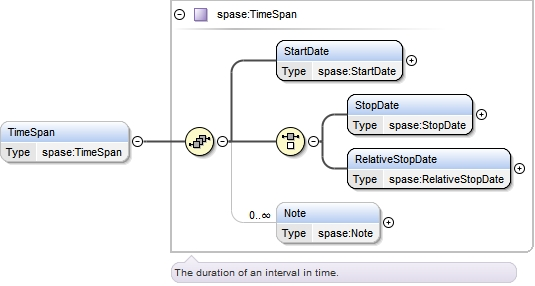Type spase:TimeSpan
Properties
 content complex minOccurs 0 maxOccurs 1
Model
Children spase:Note, spase:RelativeStopDate, spase:StartDate, spase:StopDate
Instance
 ``` {1,1} {1,1} {1,1} {0,unbounded} ```
Source
 ``
Element spase:TimeSpan / spase:StartDate
Namespace http://www.spase-group.org/data/schema
DiagramType spase:StartDate
Properties
 content simple minOccurs 1 maxOccurs 1
Source
 ``
Element spase:TimeSpan / spase:StopDate
Namespace http://www.spase-group.org/data/schema
DiagramType spase:StopDate
Properties
 content simple
Source
 ``
Element spase:TimeSpan / spase:RelativeStopDate
Namespace http://www.spase-group.org/data/schema
Diagram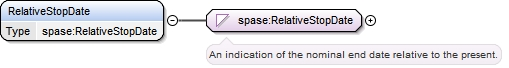Type spase:RelativeStopDate
Properties
 content simple
Source
 ``
Element spase:TimeSpan / spase:Note
Namespace http://www.spase-group.org/data/schema
DiagramType spase:Note
Properties
 content simple minOccurs 0 maxOccurs unbounded
Source
 ``
Element spase:Catalog / spase:Caveats
Namespace http://www.spase-group.org/data/schema
Diagram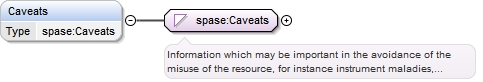Type spase:Caveats
Properties
 content simple minOccurs 0 maxOccurs 1
Source
 ``
Element spase:Catalog / spase:Keyword
Namespace http://www.spase-group.org/data/schema
DiagramType spase:Keyword
Properties
 content simple minOccurs 0 maxOccurs unbounded
Source
 ``
Element spase:Catalog / spase:InputResourceID
Namespace http://www.spase-group.org/data/schema
DiagramType spase:InputResourceID
Properties
 content simple minOccurs 0 maxOccurs unbounded
Source
 ``
Element spase:Catalog / spase:Parameter
Namespace http://www.spase-group.org/data/schema
Diagram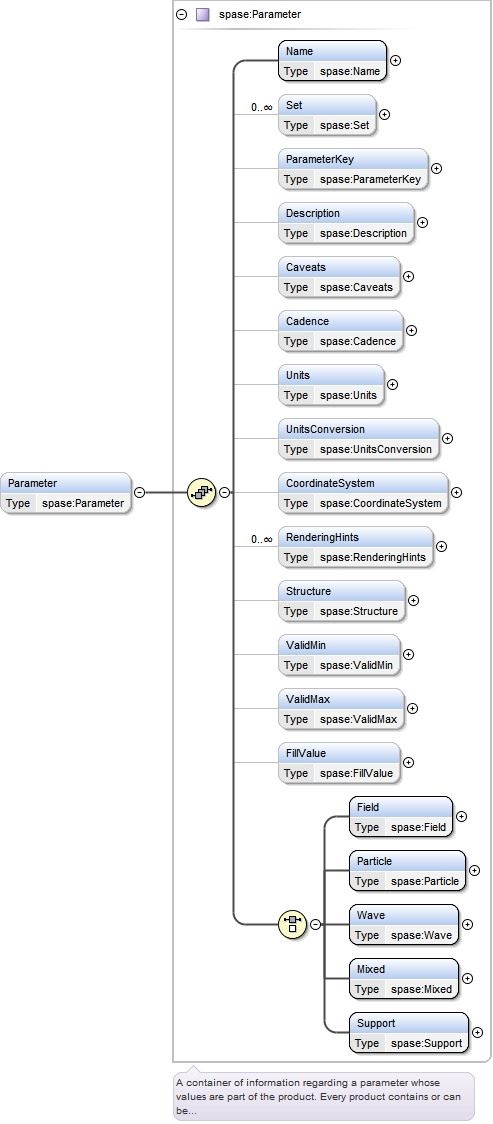Type spase:Parameter
Properties
 content complex minOccurs 0 maxOccurs unbounded
Model
Children spase:Cadence, spase:Caveats, spase:CoordinateSystem, spase:Description, spase:Field, spase:FillValue, spase:Mixed, spase:Name, spase:ParameterKey, spase:Particle, spase:RenderingHints, spase:Set, spase:Structure, spase:Support, spase:Units, spase:UnitsConversion, spase:ValidMax, spase:ValidMin, spase:Wave
Instance
 ``` {1,1} {0,unbounded} {0,1} {0,1} {0,1} {0,1} {0,1} {0,1} {0,1} {0,unbounded} {0,1} {0,1} {0,1} {0,1} {1,1} {1,1} {1,1} {1,1} {1,1} ```
Source
 ``
Element spase:Parameter / spase:Name
Namespace http://www.spase-group.org/data/schema
DiagramType spase:Name
Properties
 content simple minOccurs 1 maxOccurs 1
Source
 ``
Element spase:Parameter / spase:Set
Namespace http://www.spase-group.org/data/schema
DiagramType spase:Set
Properties
 content simple minOccurs 0 maxOccurs unbounded
Source
 ``
Element spase:Parameter / spase:ParameterKey
Namespace http://www.spase-group.org/data/schema
Diagram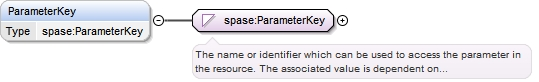Type spase:ParameterKey
Properties
 content simple minOccurs 0 maxOccurs 1
Source
 ``
Element spase:Parameter / spase:Description
Namespace http://www.spase-group.org/data/schema
DiagramType spase:Description
Properties
 content simple minOccurs 0 maxOccurs 1
Source
 ``
Element spase:Parameter / spase:Caveats
Namespace http://www.spase-group.org/data/schema
DiagramType spase:Caveats
Properties
 content simple minOccurs 0 maxOccurs 1
Source
 ``
Namespace http://www.spase-group.org/data/schema
Diagram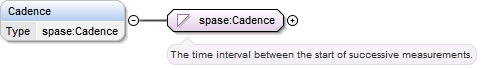Properties
 content simple minOccurs 0 maxOccurs 1
Source
 ``
Element spase:Parameter / spase:Units
Namespace http://www.spase-group.org/data/schema
Diagram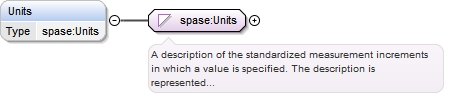Type spase:Units
Properties
 content simple minOccurs 0 maxOccurs 1
Source
 ``
Element spase:Parameter / spase:UnitsConversion
Namespace http://www.spase-group.org/data/schema
DiagramType spase:UnitsConversion
Properties
 content simple minOccurs 0 maxOccurs 1
Source
 ``
Element spase:Parameter / spase:CoordinateSystem
Namespace http://www.spase-group.org/data/schema
Diagram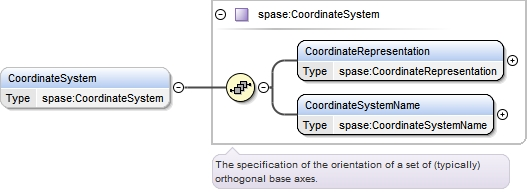Type spase:CoordinateSystem
Properties
 content complex minOccurs 0 maxOccurs 1
Model
Children spase:CoordinateRepresentation, spase:CoordinateSystemName
Instance
 ``` {1,1} {1,1} ```
Source
 ``
Element spase:CoordinateSystem / spase:CoordinateRepresentation
Namespace http://www.spase-group.org/data/schema
Diagram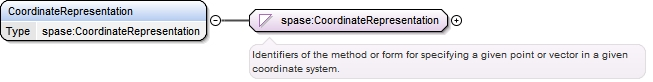Type spase:CoordinateRepresentation
Properties
 content simple minOccurs 1 maxOccurs 1
Facets
enumeration Cartesian
 `A representation in which a position vector or a measured vector (e.g., field or flow) is specified by its components along the base axes of the coordinate system.`
enumeration Cylindrical
 `A coordinate representation of a position vector or measured vector (field or flow) by its k-component, the magnitude of its projection into the i-j plane, and the azimuthal angle of the i-j plane projection.`
enumeration Spherical
 `A coordinate representation of a position vector or of a measured vector by its magnitude and two direction angles. The angles are relative to the base axes of the coordinate system used. Typically the angles are phi [azimuth angle, =arctan (j/i)] and theta, where theta may be a polar angle, arctan {[SQRT(i^2+j^2)]/k}, or an elevation angle, arctan [k/SQRT (i^2+j^2)].`
Source
 ``
Element spase:CoordinateSystem / spase:CoordinateSystemName
Namespace http://www.spase-group.org/data/schema
Diagram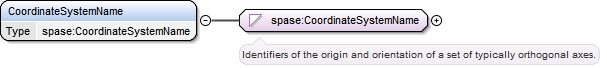Type spase:CoordinateSystemName
Properties
 content simple minOccurs 1 maxOccurs 1
Facets
enumeration CGM
 `Corrected Geomagnetic - A coordinate system from a spatial point with GEO radial distance and geomagnetic latitude and longitude, follow the epoch-appropriate IGRF/DGRF model field vector through to the point where the field line crosses the geomagnetic dipole equatorial plane. Then trace the dipole magnetic field vector Earthward from that point on the equatorial plane, in the same hemisphere as the original point, until the initial radial distance is reached. Designate the dipole latitude and longitude at that point as the CGM latitude and longitude of the original point. See `
enumeration Carrington
 `A coordinate system which is centered at the Sun and is fixed with respect to the synodic rotation rate; the mean synodic value is about 27.2753 days. The Astronomical Almanac gives a value for Carrington longitude of 349.03 degrees at 0000 UT on 1 January 1995.`
enumeration DM
 `Dipole Meridian - A coordinate system centered at the observation point. Z axis is parallel to the Earths dipole axis, positive northward. X is in the plane defined by Z and the line linking the observation point with the Earths center. Y is positive eastward. See `
enumeration GEI
 `GEI Geocentric Equatorial Inertial - A coordinate system where the Z axis is along Earths spin vector, positive northward. X axis points towards the first point of Aries (from the Earth towards the Sun at the vernal equinox). See Russell, 1971. When the X axis is the direction of the mean vernal equinox of J2000, the coordinate system is also called GCI. Then the Z axis is also defined as being normal to the mean Earth equator of J2000.`
enumeration GEO
 `Geographic - geocentric corotating - A coordinate system where the Z axis is along Earths spin vector, positive northward. X axis lies in Greenwich meridian, positive towards Greenwich. See Russell, 1971.`
enumeration GSE
 `Geocentric Solar Ecliptic - A coordinate system where the X axis is from Earth to Sun. Z axis is normal to the ecliptic, positive northward. See Russell, 1971.`
enumeration GSEQ
 `Geocentric Solar Equatorial - A coordinate system where the X axis is from Earth to Sun. Y axis is parallel to solar equatorial plane. Z axis is positive northward. See Russell, 1971`
enumeration GSM
 `Geocentric Solar Magnetospheric - A coordinate system where the X axis is from Earth to Sun, Z axis is northward in a plane containing the X axis and the geomagnetic dipole axis. See Russell, 1971`
enumeration HAE
 `Heliocentric Aries Ecliptic - A coordinate system where the Z axis is normal to the ecliptic plane, positive northward. X axis is positive towards the first point of Aries (from Earth to Sun at vernal equinox). Same as SE below. See Hapgood, 1992.`
enumeration HCC
 `Heliocentric Cartesian - A 3-D orthonormal coordinate system that is primarily intended to specify with two dimensions a point on the solar disk. The Z axis points toward the observer. The Y axis lies in the plane defined by the solar spin vector and the Z axis, positive northward. The X axis is perpendicular to the Y and Z axes, positive toward solar west. Standard representation for this system is via the points x and y values, expressed either as physical distances or as fractions of the solar disk radius.`
enumeration HCI
 `Heliographic Carrington Inertial.`
enumeration HCR
 `Heliocentric Radial - A 3-D orthonormal coordinate system that is primarily intended to specify with two dimensions a point on the solar disk. The Z axis points toward the observer. The Y axis lies in the plane defined by the solar spin vector and the Z axis, positive northward. The X axis is perpendicular to the Y and Z axes, positive toward solar west. Standard representation for this system is via the points distance rho from the Z axis [Rho = SQRT(x**2 + y**2)] and its phase angle psi measured counterclockwise from the +Y axis [psi = arctan (-y/x)]`
enumeration HEE
 `Heliocentric Earth Ecliptic - A coordinate system where the Z axis is normal to the ecliptic plane, positive northward. X axis points from Sun to Earth. See Hapgood, 1992`
enumeration HEEQ
 `Heliocentric Earth Equatorial - A coordinate system where the Z axis is normal to the solar equatorial plane, positive northward. X axis is generally Earthward in the plane defined by the Z axis and the Sun-Earth direction. See Hapgood, 1992.`
enumeration HG
 `Heliographic - A heliocentric rotating coordinate system where the Z axis is normal to the solar equatorial plane, positive northward. X, Y axes rotate with a 25.38 day period. The zero longitude (X axis) is defined as the longitude that passed through the ascending node of the solar equator on the ecliptic plane on 1 January, 1854 at 12 UT. See `
enumeration HGI
 `Heliographic Inertial - A heliocentric coordinate system where the Z axis is normal to the solar equatorial plane, positive northward. X axis is along the intersection line between solar equatorial and ecliptic planes. The X axis was positive at SE longitude of 74.367 deg on Jan 1, 1900. (See SE below.) See `
enumeration HPC
 `Helioprojective Cartesian = A 3-D orthonormal (left-handed) coordinate system that is primarily intended to specify with two dimensions a point on the solar disk. The Z axis points from the observer to the center of the solar disk. The Y axis lies in the plane defined by the solar spin vector and the Z axis, positive northward. The X axis is perpendicular to the Y and Z axes, positive toward solar west. Given as the distance between the observer and the center of the solar disk, the standard representation of an (x,y) point on the solar disk is via the points longitude angle [arctan (x/d)] and latitude angle [arctan y/d].`
enumeration HPR
 `Helioprojective Radial - A 3-D orthonormal (left-handed) coordinate system that is primarily intended to specify with two dimensions a point on the solar disk. The Z axis points from the observer to the center of the solar disk. The Y axis lies in the plane defined by the solar spin vector and the Z axis, positive northward. The X axis is perpendicular to the Y and Z axes, positive toward solar west. Given as the distance between the observer and the center of the solar disk, the standard representation for this system of an (x,y) point on the solar disk is via the points latitude angle theta {= arctan [SQRT(x**2 + y**2)]/d]} or equivalent declination parameter delta (= theta - 90 deg), and its phase angle psi as measured counter- clockwise from the +Y axis [psi = arctan (-y/x)].`
enumeration J2000
 `An astronomical coordinate system which uses the mean equator and equinox of Julian date 2451545.0 TT (Terrestrial Time), or January 1, 2000, noon TT. (aka J2000) to define a celestial reference frame.`
enumeration LGM
 `Local Geomagnetic - A coordinate system used mainly for Earth surface or near Earth surface magnetic field data. X axis northward from observation point in a geographic meridian. Z axis downward towards Earths center. In this system, H (total horizontal component) = SQRT (Bx^2 + By^2) and D (declination angle) = arctan (By/Bx)`
enumeration MAG
 `Geomagnetic - geocentric. Z axis is parallel to the geomagnetic dipole axis, positive north. X is in the plane defined by the Z axis and the Earths rotation axis. If N is a unit vector from the Earths center to the north geographic pole, the signs of the X and Y axes are given by Y = N x Z, X = Y x Z.. See Russell, 1971, and `
enumeration MFA
 `Magnetic Field Aligned - A coordinate system spacecraft-centered system with Z in the direction of the ambient magnetic field vector. X is in the plane defined by Z and the spacecraft-Sun line, positive sunward. See `
enumeration RTN
 `Radial Tangential Normal. Typically centered at a spacecraft. Used for IMF and plasma V vectors. R (radial) axis is radially away from the Sun, T (tangential) axis is normal to the plane formed by R and the Suns spin vector, positive in the direction of planetary motion. N (normal) is R x T.`
enumeration SC
 `Spacecraft - A coordinate system defined by the spacecraft geometry and/or spin. Often has Z axis parallel to spacecraft spin vector. X and Y axes may or may not corotate with the spacecraft. See SR and SR2 below.`
enumeration SE
 `Solar Ecliptic - A heliocentric coordinate system where the Z axis is normal to the ecliptic plane, positive northward. X axis is positive towards the first point of Aries (from Earth to Sun at vernal equinox). Same as HAE above. See `
enumeration SM
 `Solar Magnetic - A geocentric coordinate system where the Z axis is northward along Earths dipole axis, X axis is in plane of z axis and Earth-Sun line, positive sunward. See Russell, 1971.`
enumeration SR
 `Spin Reference - A special case of a Spacecraft (SC) coordinate system for a spinning spacecraft. Z is parallel to the spacecraft spin vector. X and Y rotate with the spacecraft. See `
enumeration SR2
 `Spin Reference 2 - A special case of a Spacecraft (SC) coordinate system for a spinning spacecraft. Z is parallel to the spacecraft spin vector. X is in the plane defined by Z and the spacecraft-Sun line, positive sunward. See `
enumeration SSE
 `Spacecraft Solar Ecliptic - A coordinate system used for deep space spacecraft, for example Helios. - X axis from spacecraft to Sun. Z axis normal to ecliptic plane, positive northward. Note: Angle between normals to ecliptic and to Helios orbit plane ~ 0.25 deg.`
enumeration SSE_L
 `Selenocentric Solar Ecliptic. The X axis points from the center of the Earths moon to the sun, the Z axis is normal to the ecliptic plane, positive northward. And the Y axis completes the right-handed set of axes.`
enumeration SpacecraftOrbitPlane
 `A coordinate system where X lies in the plane normal to and in the direction of motion of the spacecraft, Z is normal to this plane and Y completes the triad in a right-handed coordinate system.`
enumeration WGS84
 `The World Geodetic System (WGS) defines a reference frame for the earth, for use in geodesy and navigation. The WGS84 uses the zero meridian as defined by the Bureau International de lHeure.`
Source
 ``
Element spase:Parameter / spase:RenderingHints
Namespace http://www.spase-group.org/data/schema
Diagram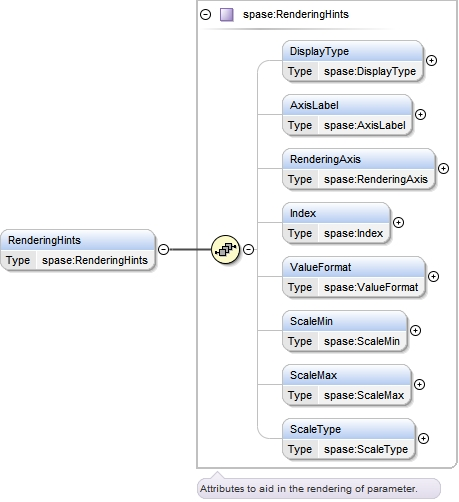Type spase:RenderingHints
Properties
 content complex minOccurs 0 maxOccurs unbounded
Model
Children spase:AxisLabel, spase:DisplayType, spase:Index, spase:RenderingAxis, spase:ScaleMax, spase:ScaleMin, spase:ScaleType, spase:ValueFormat
Instance
 ``` {0,1} {0,1} {0,1} {0,1} {0,1} {0,1} {0,1} {0,1} ```
Source
 ``
Element spase:RenderingHints / spase:DisplayType
Namespace http://www.spase-group.org/data/schema
DiagramType spase:DisplayType
Properties
 content simple minOccurs 0 maxOccurs 1
Facets
enumeration Image
 `A two-dimensional representation of data with values at each element of the array related to an intensity or a color.`
enumeration Plasmagram
 `The characterization of signal strengths in active sounding measurements as a function of virtual range or signal delay time and sounding frequency. A Plasmagram is also referred to as an Ionogram.`
enumeration Spectrogram
 `The characterization of signal strengths as a function of frequency (or energy) and time.`
enumeration StackPlot
 `A representation of data showing multiple sets of observations on a single plot, possibly offsetting each plot by some uniform amount.`
enumeration TimeSeries
 `A representation of data showing a set of observations taken at different points in time and charted as a time series.`
enumeration WaveForm
 `Spatial or temporal variations of wave amplitude over wave-period timescales.`
Source
 ``
Element spase:RenderingHints / spase:AxisLabel
Namespace http://www.spase-group.org/data/schema
DiagramType spase:AxisLabel
Properties
 content simple minOccurs 0 maxOccurs 1
Source
 ``
Element spase:RenderingHints / spase:RenderingAxis
Namespace http://www.spase-group.org/data/schema
DiagramType spase:RenderingAxis
Properties
 content simple minOccurs 0 maxOccurs 1
Facets
enumeration ColorBar
 `A spectrum or set of colors used to represent data values.`
enumeration Horizontal
 `Parallel to or in the plane of the horizon or a base line.`
enumeration Vertical
 `Perpendicular to the plane of the horizon or a base line.`
Source
 ``
Element spase:RenderingHints / spase:Index
Namespace http://www.spase-group.org/data/schema
DiagramType spase:Index
Type hierarchy
Properties
 content simple minOccurs 0 maxOccurs 1
Source
 ``
Element spase:RenderingHints / spase:ValueFormat
Namespace http://www.spase-group.org/data/schema
Diagram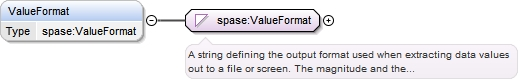Type spase:ValueFormat
Properties
 content simple minOccurs 0 maxOccurs 1
Source
 ``
Element spase:RenderingHints / spase:ScaleMin
Namespace http://www.spase-group.org/data/schema
Diagram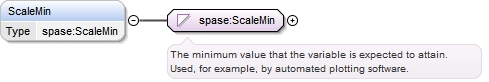Type spase:ScaleMin
Properties
 content simple minOccurs 0 maxOccurs 1
Source
 ``
Element spase:RenderingHints / spase:ScaleMax
Namespace http://www.spase-group.org/data/schema
Diagram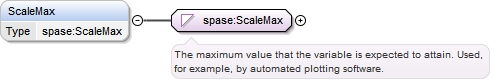Type spase:ScaleMax
Properties
 content simple minOccurs 0 maxOccurs 1
Source
 ``
Element spase:RenderingHints / spase:ScaleType
Namespace http://www.spase-group.org/data/schema
Diagram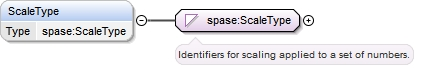Type spase:ScaleType
Properties
 content simple minOccurs 0 maxOccurs 1
Facets
enumeration LinearScale
 `Intervals which are equally spaced.`
enumeration LogScale
 `Intervals which are spaced proportionally to the logarithms of the values being represented.`
Source
 ``
Element spase:Parameter / spase:Structure
Namespace http://www.spase-group.org/data/schema
Diagram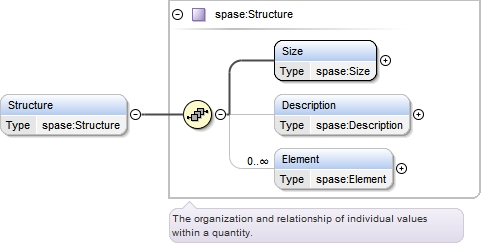Type spase:Structure
Properties
 content complex minOccurs 0 maxOccurs 1
Model
Children spase:Description, spase:Element, spase:Size
Instance
 ``` {1,1} {0,1} {0,unbounded} ```
Source
 ``
Element spase:Structure / spase:Size
Namespace http://www.spase-group.org/data/schema
Diagram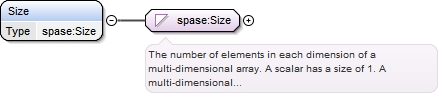Type spase:Size
Type hierarchy
Properties
 content simple minOccurs 1 maxOccurs 1
Source
 ``
Element spase:Structure / spase:Description
Namespace http://www.spase-group.org/data/schema
DiagramType spase:Description
Properties
 content simple minOccurs 0 maxOccurs 1
Source
 ``
Element spase:Structure / spase:Element
Namespace http://www.spase-group.org/data/schema
Diagram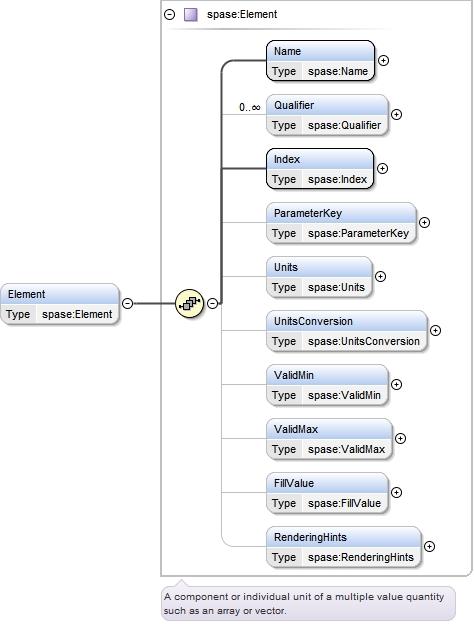Type spase:Element
Properties
 content complex minOccurs 0 maxOccurs unbounded
Model
Children spase:FillValue, spase:Index, spase:Name, spase:ParameterKey, spase:Qualifier, spase:RenderingHints, spase:Units, spase:UnitsConversion, spase:ValidMax, spase:ValidMin
Instance
 ``` {1,1} {0,unbounded} {1,1} {0,1} {0,1} {0,1} {0,1} {0,1} {0,1} {0,1} ```
Source
 ``
Element spase:Element / spase:Name
Namespace http://www.spase-group.org/data/schema
DiagramType spase:Name
Properties
 content simple minOccurs 1 maxOccurs 1
Source
 ``
Element spase:Element / spase:Qualifier
Namespace http://www.spase-group.org/data/schema
DiagramType spase:Qualifier
Properties
 content simple minOccurs 0 maxOccurs unbounded
Facets
enumeration Anisotropy
 `Direction-dependent property.`
enumeration Array
 `A sequence of values corresponding to the elements in a rectilinear, n-dimension matrix. Each value can be referenced by a unique index.`
enumeration Average
 `The statistical mean; the sum of a set of values divided by the number of values in the set.`
enumeration Characteristic
 `A quantity which can be easily identified and measured in a given environment.`
enumeration Circular
 `Relative to polarization, right-hand circularly polarized light is defined such that the electric field is rotating clockwise as seen by an observer towards whom the wave is moving. Left-hand circularly polarized light is defined such that the electric field is rotating counterclockwise as seen by an observer towards whom the wave is moving. The polarization of magnetohydrodynamic waves is specified with respect to the ambient mean magnetic field : right-hand polarized waves have a transverse electric field component which turns in a right-handed sense (that of the gyrating electrons) around the magnetic field.`
enumeration Column
 `A two-dimensional measure of a quantity. The column is the area over which the quantity is measured.`
enumeration Component
 `Projection of a vector along one of the base axes of a coordinate system.`
enumeration Component.I
 `Projection of a vector along the first named axis of a coordinate system. Typically the X axis, but could be the R axis for an RTN coordinate system.`
enumeration Component.J
 `Projection of a vector along the second named axis of a coordinate system. Typically the Y axis, but could be the T axis for an RTN coordinate system.`
enumeration Component.K
 `Projection of a vector along the third named axis of a coordinate system. Typically the Z axis, but could be the N axis for an RTN coordinate system.`
enumeration Core
 `The central or main part of an object or calculated distribution. For example, the part of a distribution of particles at low energies that is a thermal (Maxwellian) population.`
enumeration CrossSpectrum
 `The Fourier transform of the cross correlation of two physical or empirical observations.`
enumeration Deviation
 `The difference between an observed value and the expected value of a quantity.`
enumeration Differential
 `A measurement within a narrow range of energy and/or solid angle.`
enumeration Direction
 `The spatial relation between an object and another object, the orientation of the object or the course along which the object points or moves.`
enumeration DirectionAngle
 `The angle between a position vector or measured vector (or one of its projections onto a plane) and one of the base axes of the coordinate system.`
enumeration DirectionAngle.AzimuthAngle
 `The angle between the projection into the i-j plane of a position or measured vector and the i-axis of the coordinate system. Mathematically defined as arctan(j/i). This term could be also applied to angles measured in different planes, for example the IMF clock angle defined as arctan(`
enumeration DirectionAngle.ElevationAngle
 `The angle between the position or measured vector and the i-j plane of the coordinate system. Mathematically defined as arctan(k/SQRT(i^2+j^2)).`
enumeration DirectionAngle.PolarAngle
 `The angle between the position or measured vector and the k-axis of the coordinate system. Mathematically defined as arctan([SQRT(i^2+j^2)]/k). This term could be also applied to angles between the vector and other components, for example the IMF cone angle defined as arccos(Bx/Bt).`
enumeration Directional
 `A measurement within a narrow range of solid angle.`
enumeration FieldAligned
 `The component of a quantity which is oriented in the same direction of a field.`
enumeration Fit
 `Values that make an model agree with the data.`
enumeration Group
 `An assemblage of values that a certain relation or common characteristic.`
enumeration Halo
 `The part of an object or distribution surrounding some central body or distribution. For example, the particles above the core energies that show enhancements above the thermal population. Typically, a power law tail shows a break from the core Maxwellian at a particular energy.`
enumeration Integral
 `A flux measurement in a broad range of energy and solid angle.`
enumeration Integral.Area
 `Integration over the extent of a planar region, or of the surface of a solid.`
enumeration Integral.Bandwidth
 `Integration over the width a frequency band.`
enumeration Integral.SolidAngle
 `Integration over the angle in three-dimensional space that an object subtends at a point.`
enumeration LineOfSight
 `The line of sight is the line that connects the observer with the observed object. This expression is often used with measurements of Doppler velocity and magnetic field in magnetograms, where only the component of the vector field directed along the line of sight is measured.`
enumeration Linear
 `Polarization where the E-field vector is confined to a given plane`
enumeration Magnitude
 `A measure of the strength of a vector quantity or length of its representational vector.`
enumeration Maximum
 `The largest value of a batch or sample or the upper bound of a probability distribution.`
enumeration Median
 `The measure of central tendency of a set of n. values computed by ordering the values and taking the value at position (n. + 1) / 2 when n. is odd or the arithmetic mean of the values at positions n. / 2 and (n. / 2) + 1 when n. is even.`
enumeration Minimum
 `The smallest value of a batch or sample or the lower bound of a probability distribution.`
enumeration Moment
 `Parameters determined by integration over a distribution function convolved with a power of velocity.`
enumeration Parallel
 `Having the same direction as a given direction`
enumeration Peak
 `The maximum value for the quantity in question, over a period of time which is usually equal to the cadence.`
enumeration Perpendicular
 `At right angles to a given direction.`
enumeration Perturbation
 `Variations in the state of a system.`
enumeration Phase
 `A point or portion in a recurring series of changes.`
enumeration PhaseAngle
 `Phase difference between two or more waves, normally expressed in degrees.`
enumeration Projection
 `A measure of the length of a position or measured vector as projected into a plane of the coordinate system.`
enumeration Projection.IJ
 `A measure of the length of a position or measured vector projected into the i-j (typically X-Y) plane of the coordinate system.`
enumeration Projection.IK
 `A measure of the length of a position or measured vector projected into the i-k (typically X-Z) plane of the coordinate system.`
enumeration Projection.JK
 `A measure of the length of a position or measured vector projected into the j-k (typically Y-Z) plane of the coordinate system.`
enumeration Pseudo
 `Similar to or having the appearance of something else. Can be used to indicate an estimation or approximation of a particular quantity.`
enumeration Ratio
 `The relative magnitudes of two quantities.`
enumeration Scalar
 `A quantity that is completely specified by its magnitude and has no direction.`
enumeration Spectral
 `Characterized as a range or continuum of frequencies`
enumeration StandardDeviation
 `The square root of the average of the squares of deviations about the mean of a set of data. Standard deviation is a statistical measure of spread or variability.`
enumeration StokesParameters
 `A set of four parameters (usually called I,Q, U and V) which describe the polarization state of an electromagnetic wave propagating through space.`
enumeration Strahl
 `A distribution of particles concentrated in a narrow energy band. The band may be may be aligned with a secondary feature. For example, it may occur in a narrow cone aligned with the mean magnetic field direction.`
enumeration Superhalo
 `The part of an object or distribution surrounding some central body or distribution evident in a second break in the distribution function (e.g., a different power law). It consists of a population at a higher energies than for a halo.`
enumeration Symmetric
 `Equal distribution about one or more axes.`
enumeration Tensor
 `A generalized linear quantity or geometrical entity that can be expressed as a multi-dimensional array relative to a choice of basis of the particular space on which it is defined.`
enumeration Total
 `The summation of quantities over all possible species.`
enumeration Trace
 `The sum of the elements on the main diagonal (the diagonal from the upper left to the lower right) of a square matrix.`
enumeration Uncertainty
 `A statistically defined discrepancy between a measured quantity and the true value of that quantity that cannot be corrected by calculation or calibration.`
enumeration Variance
 `A measure of dispersion of a set of data points around their mean value. The expectation value of the squared deviations from the mean.`
enumeration Vector
 `A set of parameter values each along some independent variable (e.g., components of a field in three orthogonal spatial directions; atmospheric temperature values at several altitudes, or at a given latitude and longitude;).`
Source
 ``
Element spase:Element / spase:Index
Namespace http://www.spase-group.org/data/schema
DiagramType spase:Index
Type hierarchy
Properties
 content simple minOccurs 1 maxOccurs 1
Source
 ``
Element spase:Element / spase:ParameterKey
Namespace http://www.spase-group.org/data/schema
DiagramType spase:ParameterKey
Properties
 content simple minOccurs 0 maxOccurs 1
Source
 ``
Element spase:Element / spase:Units
Namespace http://www.spase-group.org/data/schema
DiagramType spase:Units
Properties
 content simple minOccurs 0 maxOccurs 1
Source
 ``
Element spase:Element / spase:UnitsConversion
Namespace http://www.spase-group.org/data/schema
DiagramType spase:UnitsConversion
Properties
 content simple minOccurs 0 maxOccurs 1
Source
 ``
Element spase:Element / spase:ValidMin
Namespace http://www.spase-group.org/data/schema
Diagram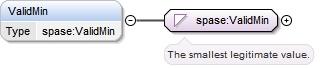Type spase:ValidMin
Properties
 content simple minOccurs 0 maxOccurs 1
Source
 ``
Element spase:Element / spase:ValidMax
Namespace http://www.spase-group.org/data/schema
Diagram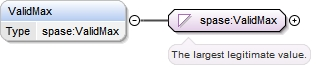Type spase:ValidMax
Properties
 content simple minOccurs 0 maxOccurs 1
Source
 ``
Element spase:Element / spase:FillValue
Namespace http://www.spase-group.org/data/schema
DiagramType spase:FillValue
Properties
 content simple minOccurs 0 maxOccurs 1
Source
 ``
Element spase:Element / spase:RenderingHints
Namespace http://www.spase-group.org/data/schema
DiagramType spase:RenderingHints
Properties
 content complex minOccurs 0 maxOccurs 1
Model
Children spase:AxisLabel, spase:DisplayType, spase:Index, spase:RenderingAxis, spase:ScaleMax, spase:ScaleMin, spase:ScaleType, spase:ValueFormat
Instance
 ``` {0,1} {0,1} {0,1} {0,1} {0,1} {0,1} {0,1} {0,1} ```
Source
 ``
Element spase:Parameter / spase:ValidMin
Namespace http://www.spase-group.org/data/schema
DiagramType spase:ValidMin
Properties
 content simple minOccurs 0 maxOccurs 1
Source
 ``
Element spase:Parameter / spase:ValidMax
Namespace http://www.spase-group.org/data/schema
DiagramType spase:ValidMax
Properties
 content simple minOccurs 0 maxOccurs 1
Source
 ``
Element spase:Parameter / spase:FillValue
Namespace http://www.spase-group.org/data/schema
DiagramType spase:FillValue
Properties
 content simple minOccurs 0 maxOccurs 1
Source
 ``
Element spase:Parameter / spase:Field
Namespace http://www.spase-group.org/data/schema
Diagram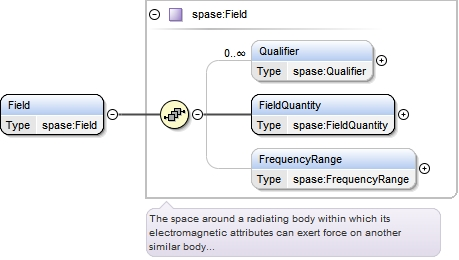Type spase:Field
Properties
 content complex
Model
Children spase:FieldQuantity, spase:FrequencyRange, spase:Qualifier
Instance
 ``` {0,unbounded} {1,1} {0,1} ```
Source
 ``
Element spase:Field / spase:Qualifier
Namespace http://www.spase-group.org/data/schema
DiagramType spase:Qualifier
Properties
 content simple minOccurs 0 maxOccurs unbounded
Facets
enumeration Anisotropy
 `Direction-dependent property.`
enumeration Array
 `A sequence of values corresponding to the elements in a rectilinear, n-dimension matrix. Each value can be referenced by a unique index.`
enumeration Average
 `The statistical mean; the sum of a set of values divided by the number of values in the set.`
enumeration Characteristic
 `A quantity which can be easily identified and measured in a given environment.`
enumeration Circular
 `Relative to polarization, right-hand circularly polarized light is defined such that the electric field is rotating clockwise as seen by an observer towards whom the wave is moving. Left-hand circularly polarized light is defined such that the electric field is rotating counterclockwise as seen by an observer towards whom the wave is moving. The polarization of magnetohydrodynamic waves is specified with respect to the ambient mean magnetic field : right-hand polarized waves have a transverse electric field component which turns in a right-handed sense (that of the gyrating electrons) around the magnetic field.`
enumeration Column
 `A two-dimensional measure of a quantity. The column is the area over which the quantity is measured.`
enumeration Component
 `Projection of a vector along one of the base axes of a coordinate system.`
enumeration Component.I
 `Projection of a vector along the first named axis of a coordinate system. Typically the X axis, but could be the R axis for an RTN coordinate system.`
enumeration Component.J
 `Projection of a vector along the second named axis of a coordinate system. Typically the Y axis, but could be the T axis for an RTN coordinate system.`
enumeration Component.K
 `Projection of a vector along the third named axis of a coordinate system. Typically the Z axis, but could be the N axis for an RTN coordinate system.`
enumeration Core
 `The central or main part of an object or calculated distribution. For example, the part of a distribution of particles at low energies that is a thermal (Maxwellian) population.`
enumeration CrossSpectrum
 `The Fourier transform of the cross correlation of two physical or empirical observations.`
enumeration Deviation
 `The difference between an observed value and the expected value of a quantity.`
enumeration Differential
 `A measurement within a narrow range of energy and/or solid angle.`
enumeration Direction
 `The spatial relation between an object and another object, the orientation of the object or the course along which the object points or moves.`
enumeration DirectionAngle
 `The angle between a position vector or measured vector (or one of its projections onto a plane) and one of the base axes of the coordinate system.`
enumeration DirectionAngle.AzimuthAngle
 `The angle between the projection into the i-j plane of a position or measured vector and the i-axis of the coordinate system. Mathematically defined as arctan(j/i). This term could be also applied to angles measured in different planes, for example the IMF clock angle defined as arctan(`
enumeration DirectionAngle.ElevationAngle
 `The angle between the position or measured vector and the i-j plane of the coordinate system. Mathematically defined as arctan(k/SQRT(i^2+j^2)).`
enumeration DirectionAngle.PolarAngle
 `The angle between the position or measured vector and the k-axis of the coordinate system. Mathematically defined as arctan([SQRT(i^2+j^2)]/k). This term could be also applied to angles between the vector and other components, for example the IMF cone angle defined as arccos(Bx/Bt).`
enumeration Directional
 `A measurement within a narrow range of solid angle.`
enumeration FieldAligned
 `The component of a quantity which is oriented in the same direction of a field.`
enumeration Fit
 `Values that make an model agree with the data.`
enumeration Group
 `An assemblage of values that a certain relation or common characteristic.`
enumeration Halo
 `The part of an object or distribution surrounding some central body or distribution. For example, the particles above the core energies that show enhancements above the thermal population. Typically, a power law tail shows a break from the core Maxwellian at a particular energy.`
enumeration Integral
 `A flux measurement in a broad range of energy and solid angle.`
enumeration Integral.Area
 `Integration over the extent of a planar region, or of the surface of a solid.`
enumeration Integral.Bandwidth
 `Integration over the width a frequency band.`
enumeration Integral.SolidAngle
 `Integration over the angle in three-dimensional space that an object subtends at a point.`
enumeration LineOfSight
 `The line of sight is the line that connects the observer with the observed object. This expression is often used with measurements of Doppler velocity and magnetic field in magnetograms, where only the component of the vector field directed along the line of sight is measured.`
enumeration Linear
 `Polarization where the E-field vector is confined to a given plane`
enumeration Magnitude
 `A measure of the strength of a vector quantity or length of its representational vector.`
enumeration Maximum
 `The largest value of a batch or sample or the upper bound of a probability distribution.`
enumeration Median
 `The measure of central tendency of a set of n. values computed by ordering the values and taking the value at position (n. + 1) / 2 when n. is odd or the arithmetic mean of the values at positions n. / 2 and (n. / 2) + 1 when n. is even.`
enumeration Minimum
 `The smallest value of a batch or sample or the lower bound of a probability distribution.`
enumeration Moment
 `Parameters determined by integration over a distribution function convolved with a power of velocity.`
enumeration Parallel
 `Having the same direction as a given direction`
enumeration Peak
 `The maximum value for the quantity in question, over a period of time which is usually equal to the cadence.`
enumeration Perpendicular
 `At right angles to a given direction.`
enumeration Perturbation
 `Variations in the state of a system.`
enumeration Phase
 `A point or portion in a recurring series of changes.`
enumeration PhaseAngle
 `Phase difference between two or more waves, normally expressed in degrees.`
enumeration Projection
 `A measure of the length of a position or measured vector as projected into a plane of the coordinate system.`
enumeration Projection.IJ
 `A measure of the length of a position or measured vector projected into the i-j (typically X-Y) plane of the coordinate system.`
enumeration Projection.IK
 `A measure of the length of a position or measured vector projected into the i-k (typically X-Z) plane of the coordinate system.`
enumeration Projection.JK
 `A measure of the length of a position or measured vector projected into the j-k (typically Y-Z) plane of the coordinate system.`
enumeration Pseudo
 `Similar to or having the appearance of something else. Can be used to indicate an estimation or approximation of a particular quantity.`
enumeration Ratio
 `The relative magnitudes of two quantities.`
enumeration Scalar
 `A quantity that is completely specified by its magnitude and has no direction.`
enumeration Spectral
 `Characterized as a range or continuum of frequencies`
enumeration StandardDeviation
 `The square root of the average of the squares of deviations about the mean of a set of data. Standard deviation is a statistical measure of spread or variability.`
enumeration StokesParameters
 `A set of four parameters (usually called I,Q, U and V) which describe the polarization state of an electromagnetic wave propagating through space.`
enumeration Strahl
 `A distribution of particles concentrated in a narrow energy band. The band may be may be aligned with a secondary feature. For example, it may occur in a narrow cone aligned with the mean magnetic field direction.`
enumeration Superhalo
 `The part of an object or distribution surrounding some central body or distribution evident in a second break in the distribution function (e.g., a different power law). It consists of a population at a higher energies than for a halo.`
enumeration Symmetric
 `Equal distribution about one or more axes.`
enumeration Tensor
 `A generalized linear quantity or geometrical entity that can be expressed as a multi-dimensional array relative to a choice of basis of the particular space on which it is defined.`
enumeration Total
 `The summation of quantities over all possible species.`
enumeration Trace
 `The sum of the elements on the main diagonal (the diagonal from the upper left to the lower right) of a square matrix.`
enumeration Uncertainty
 `A statistically defined discrepancy between a measured quantity and the true value of that quantity that cannot be corrected by calculation or calibration.`
enumeration Variance
 `A measure of dispersion of a set of data points around their mean value. The expectation value of the squared deviations from the mean.`
enumeration Vector
 `A set of parameter values each along some independent variable (e.g., components of a field in three orthogonal spatial directions; atmospheric temperature values at several altitudes, or at a given latitude and longitude;).`
Source
 ``
Element spase:Field / spase:FieldQuantity
Namespace http://www.spase-group.org/data/schema
Diagram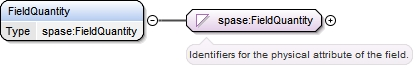Type spase:FieldQuantity
Properties
 content simple minOccurs 1 maxOccurs 1
Facets
enumeration Current
 `The flow of electrons through a conductor caused by a potential difference.`
enumeration Electric
 `The physical attribute that exerts an electrical force.`
enumeration Electromagnetic
 `Electric and magnetic field variations in time and space that propagate through a medium or a vacuum with the waves propagation, electric field, and magnetic field vectors forming an orthogonal triad. Waves in this category are detected by having their field quantities measured.`
enumeration Gyrofrequency
 `The number of gyrations around a magnetic guiding center (field line) a charged particle makes per unit time due to the Lorentz force.`
enumeration Magnetic
 `The physical attribute attributed to a magnet or its equivalent.`
enumeration PlasmaFrequency
 `A number-density-dependent characteristic frequency of a plasma.`
enumeration Potential
 `The work required per unit charge to move a charge from a reference point to a point at infinity (electric potential is defined to be zero). The electric potential of a spacecraft is often referred to as the spacecraft potential. The spacecraft potential is the electric potential of the spacecraft relative to the potential of the nearby plasma. The spacecraft potential is non-zero because the spacecraft charges to the level that the emitted photoelectron flux going to infinity is balanced by the plasma electron flux to the spacecraft.`
enumeration PoyntingFlux
 `Electromagnetic energy flux transported by a wave characterized as the rate of energy transport per unit area per steradian.`
Source
 ``
Element spase:Field / spase:FrequencyRange
Namespace http://www.spase-group.org/data/schema
Diagram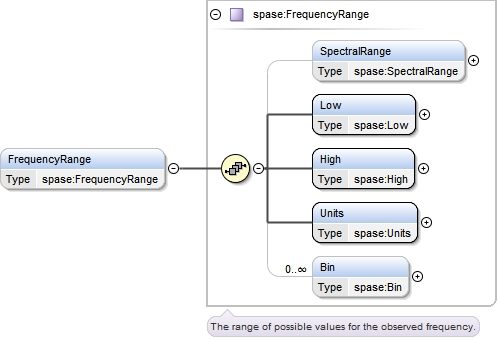Type spase:FrequencyRange
Properties
 content complex minOccurs 0 maxOccurs 1
Model
Children spase:Bin, spase:High, spase:Low, spase:SpectralRange, spase:Units
Instance
 ``` {0,1} {1,1} {1,1} {1,1} {0,unbounded} ```
Source
 ``
Element spase:FrequencyRange / spase:SpectralRange
Namespace http://www.spase-group.org/data/schema
Diagram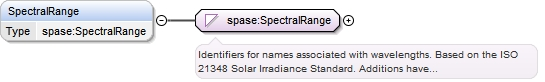Type spase:SpectralRange
Properties
 content simple minOccurs 0 maxOccurs 1
Facets
enumeration CaK
 `A spectrum with a wavelength of range centered near 393.5 nm. VSO nickname: Ca-K image with range of 391.9 nm to 395.2 nm.`
enumeration ExtremeUltraviolet
 `A spectrum with a wavelength range of 10.0 nm to 125.0nm. VSO nickname: EUV image with a range of of 10.0 nm to 125.0 nm`
enumeration FarUltraviolet
 `A spectrum with a wavelength range of 122 nm to 200.0nm. VSO nickname: FUV image with a range of 122.0 nm to 200 nm`
enumeration GammaRays
 `Photons with a wavelength range: 0.00001 to 0.001 nm`
enumeration Halpha
 `A spectrum with a wavelength range centered at 656.3 nm. VSO nickname: H-alpha image with a spectrum range of of 655.8 nm to 656.8 nm.`
enumeration HardXrays
 `Photons with a wavelength range: 0.001 to 0.1 nm and an energy range of 12 keV to 120 keV`
enumeration He10830
 `A spectrum with a wavelength range centered at 1082.9 nm. VSO nickname: He 10830 image with a range of 1082.5 nm to 1083.3 nm.`
enumeration He304
 `A spectrum centered around the resonance line of ionised helium at 304 Angstrom (30.4 nm).`
enumeration Infrared
 `Photons with a wavelength range: 760 to 1.00x10^6 nm`
enumeration K7699
 `A spectrum with a wavelength range centred at 769.9 nm. VSO nickname: K-7699 dopplergram with a range of 769.8 nm to 770.0 nm.`
enumeration LBHBand
 `Lyman-Birge-Hopfield band in the far ultraviolet range with wavelength range of 140nm to 170 nm.`
enumeration Microwave
 `Photons with a wavelength range: 1.00x10^6 to 1.50x10^7 nm`
 `A spectrum with a wavelength range of centered at 589.3 nm. VSO nickname: Na-D image with a range of 588.8 nm to 589.8 nm.`
enumeration Ni6768
 `A spectrum with a wavelength range centered at 676.8 nm. VSO nickname: Ni-6768 dopplergram with a range of of 676.7 nm to 676.9 nm.`
enumeration Optical
 `Photons with a wavelength range: 380 to 760 nm`
 `Photons with a wavelength range: 100,000 to 1.00x10^11 nm`
 `X-Rays with an energy range of 0.12 keV to 12 keV.`
 `Photons with a wavelength range: 10 to 400 nm.`
 `Photons with a wavelength in the visible range for humans.`
 `Photons with a wavelength range: 0.001 <= x < 10 nm`
 `